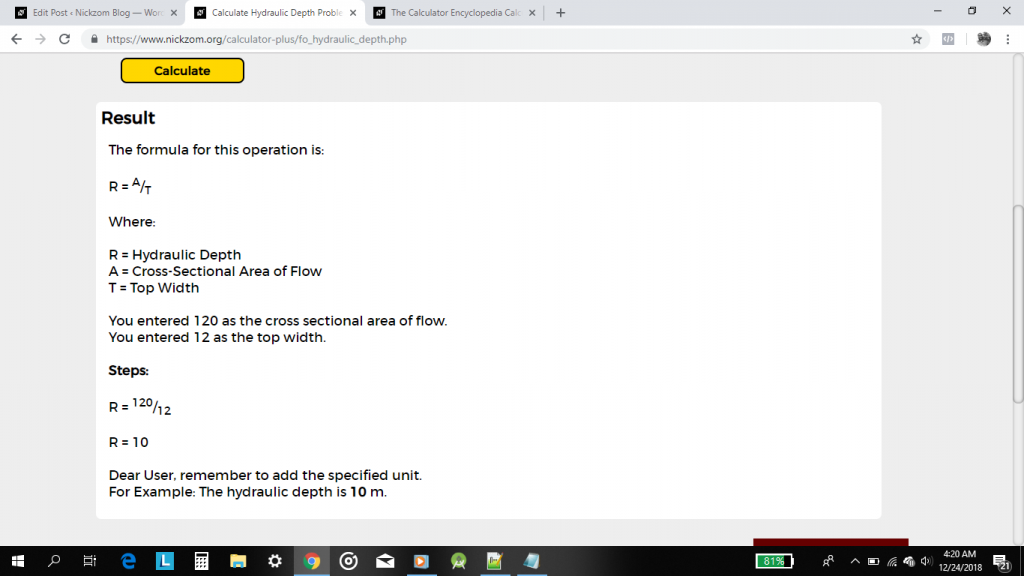# Nickzom Calculator Calculates the Hydraulic Depth of Flow in Open Channels | Fluid Mechanics

Hydraulic depth can be defined as the cross-sectional area of flow divided by the top width of surface.

Hydraulic depth is a very crucial parameter in calculations dealing with fluid mechanics and flow in open channels.

The formula for computing hydraulic depth is:

R = A / T

Where:
R = Hydraulic Depth
A = Cross-sectional Area of Flow
T =  Top Width or Top Surface Water Width

For Example:

Find the hydraulic depth of flow in an open channel where the cross-sectional area is 120 m2 and the top width is 12 m.

From the example we can see that the cross-sectional area is 120 m2 and the top width is 12 m.

R = 120 / 12
R = 10

Therefore, the hydraulic depth is 10 m.

Now, I would love to show you how to use Nickzom Calculator The Calculator Encyclopedia to get answers for your questions on hydraulic depth.

To have access to the calculator encyclopedia – Nickzom Calculator click on any of these links that suits best:
Web – https://www.nickzom.org/calculator-plus
Apple (Paid) – https://itunes.apple.com/us/app/nickzom-calculator/id1331162702?mt=8

it is preferable you obtain the professional version because that is what we are using in Nickzom Blog to showcase the powers of the calculator encyclopedia. Once you have got access to the app, proceed to the Calculator Map, then click on Mechanical under the Engineering section.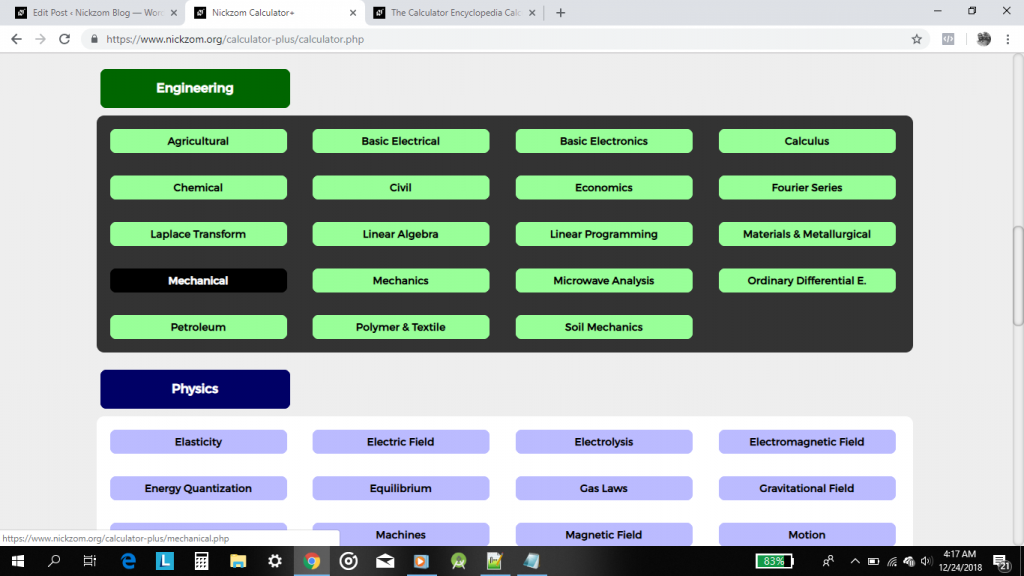Now, click on Fluid MechanicsNow, click on Flow in Open ChannelsNow, click on Hydraulic Depth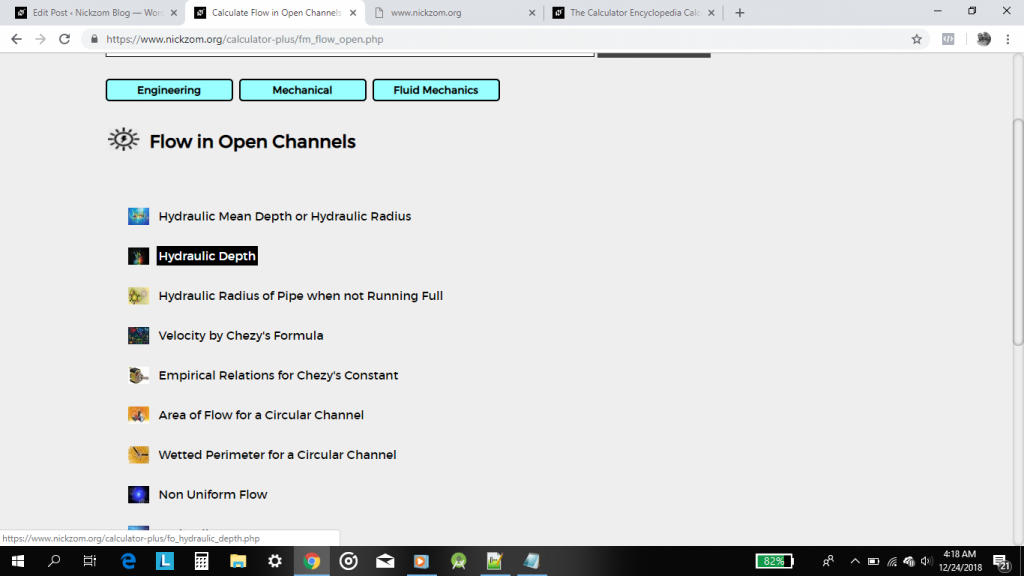A display of the parameters and input box needed to enter the values for getting the value of the hydraulic depth is now shown to you. Screenshot below!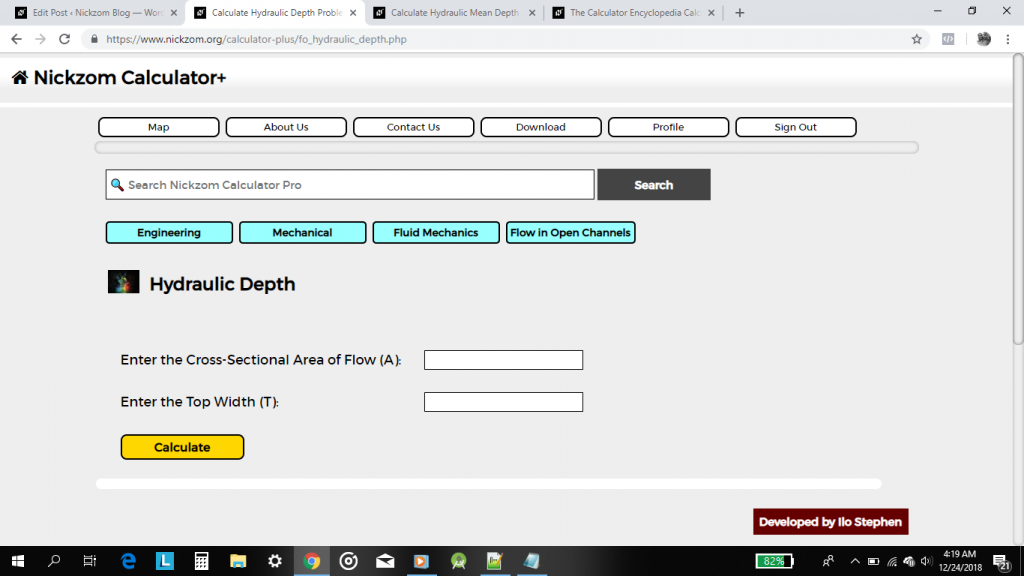From the example, the cross-sectional area of flow is 120 m2 and the top width is 12 m.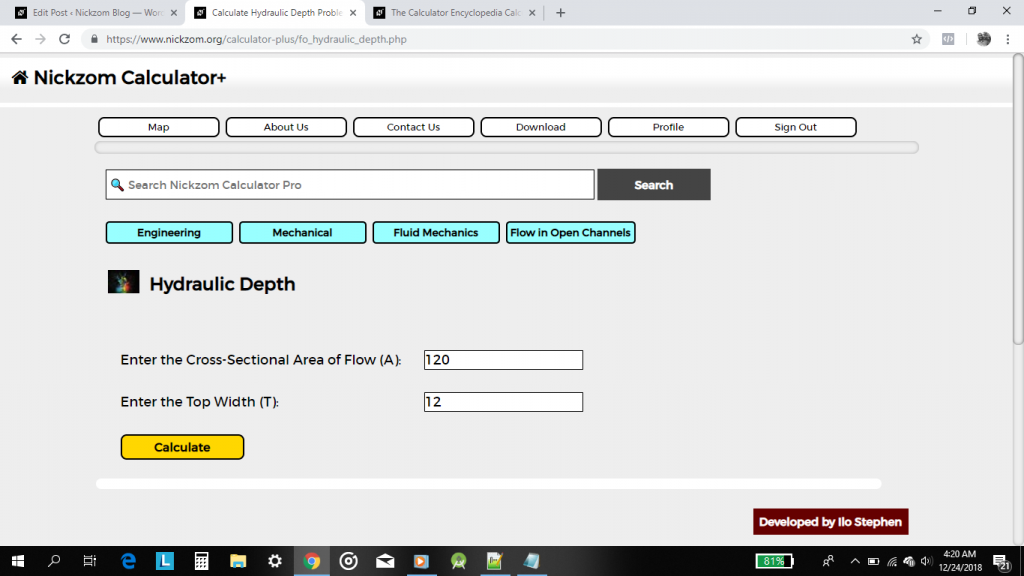Lastly, click on the Calculate button to get the hydraulic depth alongside the formula and workings. Screenshot Below!